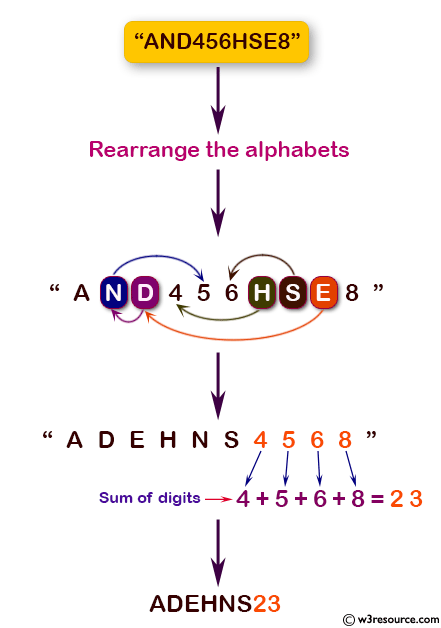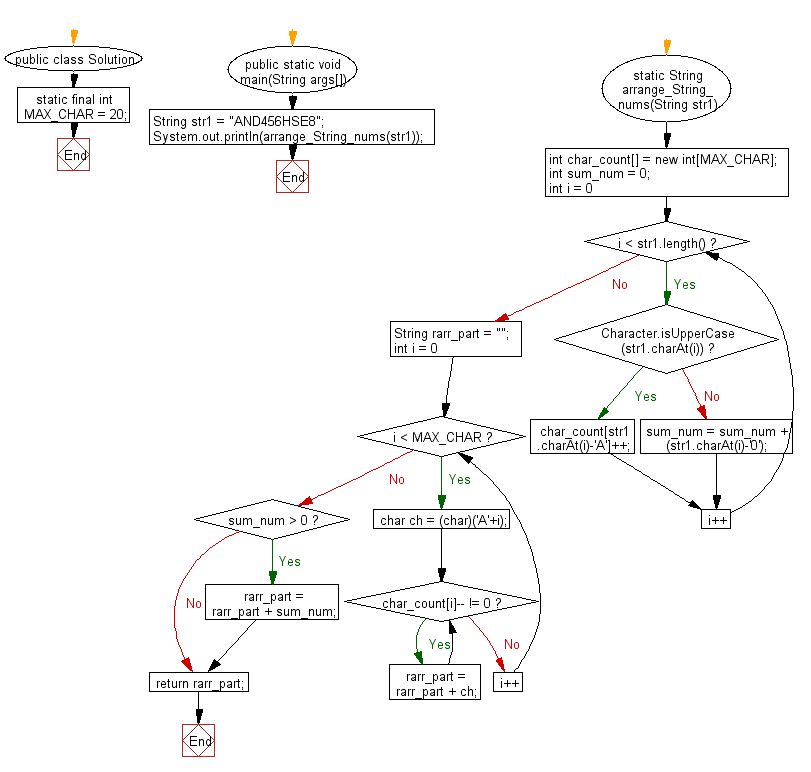﻿ Java programming exercises: Rearrange the alphabets in the order followed by the sum of digits - w3resource# Java Exercises: Rearrange the alphabets in the order followed by the sum of digits

## Java Basic: Exercise-192 with Solution

Write a Java program to rearrange the alphabets in the order followed by the sum of digits in a given string containing uppercase alphabets and integer digits (from 0 to 9).

Pictorial Presentation:Sample Solution:

Java Code:

``````import java.util.*;
import java.lang.*;
public class Solution {
static final int MAX_CHAR = 20;

public static void main(String args[]) {
String str1 = "AND456HSE8";
System.out.println(arrange_String_nums(str1));
}
static String arrange_String_nums(String str1) {
int char_count[] = new int[MAX_CHAR];
int sum_num = 0;
for (int i = 0; i < str1.length(); i++) {
if (Character.isUpperCase(str1.charAt(i)))
char_count[str1.charAt(i) - 'A']++;
else
sum_num = sum_num + (str1.charAt(i) - '0');
}

String rarr_part = "";

for (int i = 0; i < MAX_CHAR; i++) {
char ch = (char)('A' + i);
while (char_count[i]-- != 0)
rarr_part = rarr_part + ch;
}

if (sum_num > 0)
rarr_part = rarr_part + sum_num;
return rarr_part;
}
}
```
```

Sample Output:

```ADEHNS23
```

Flowchart:Java Code Editor:

Company:

What is the difficulty level of this exercise?

﻿

## Java: Tips of the Day

Parsing dates:

```import java.io.*;
import java.util.*;
import java.text.*;

String s = "2001/09/23 14:39";

SimpleDateFormat formatter = new SimpleDateFormat ("yyyy/MM/dd H:mm");
Date d = formatter.parse(s, new ParsePosition(0));
```